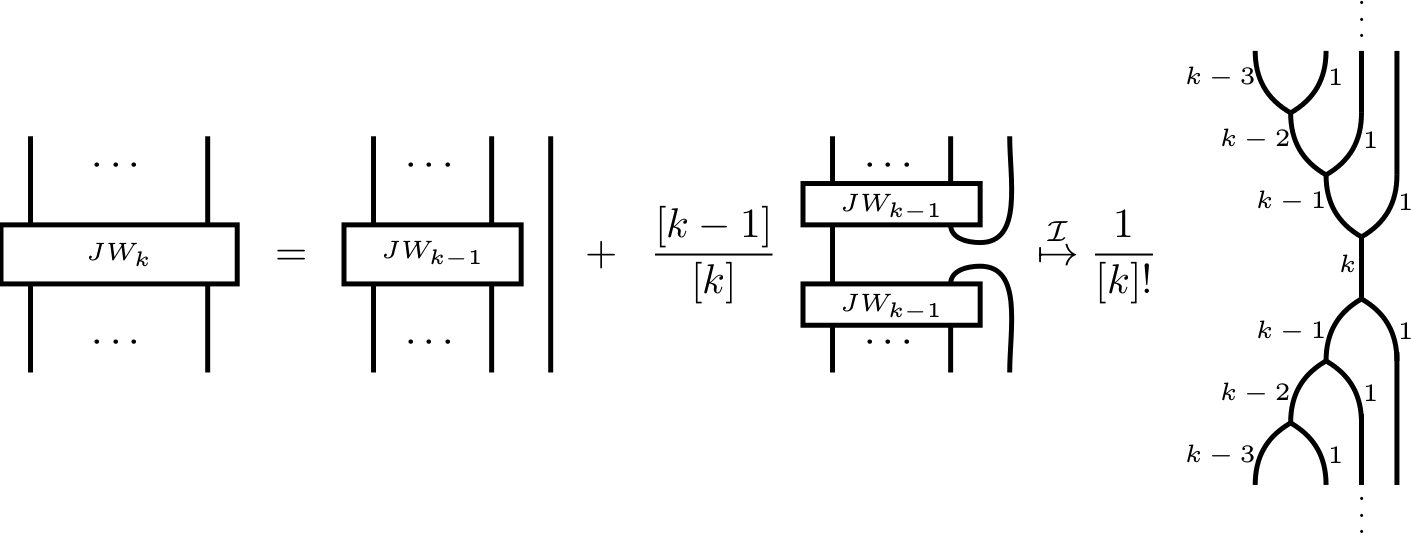## Abstract

We define and study the category of symmetric $\mathfrak{sl}_2$-webs. This category is a combinatorial description of the category of all finite dimensional quantum $\mathfrak{sl}_2$-modules. Explicitly, we show that (the additive closure of) the symmetric $\mathfrak{sl}_2$-spider is (braided monoidally) equivalent to the latter. Our main tool is a quantum version of symmetric Howe duality. As a corollary of our construction, we provide new insight into Jones-Wenzl projectors and the colored Jones polynomials.

## A few extra words

A classical result of Rumer, Teller and Weyl, modernly interpreted, states that the so-called Temperley-Lieb category $\mathcal{TL}$ describes the full subcategory of quantum $\mathfrak{sl}_2$-modules generated by tensor products of the $2$-dimensional vector representation $V$ of quantum $\mathfrak{sl}_2$.
By Karoubi completion, we get the whole category of finite dimensional $\mathfrak{sl}_2$-modules. Thus, it is a striking question if one can give a diagrammatic description of $\mathbf{KAR}(\mathcal{TL})$ as well.
We provide a new diagrammatic description of the entire category of finite dimensional quantum $\mathfrak{sl}_2$-modules.
To this end, we introduced our new description of the representation theory of quantum $\mathfrak{sl}_2$, the category of symmetric $\mathfrak{sl}_2$-webs akin to the category of “usual” $\mathfrak{sl}_n$-webs studied by many people (recall that these “usual” $\mathfrak{sl}_n$-webs give a diagrammatic presentation of the full subcategory of all finite dimensional $\mathfrak{sl}_n$-modules whose objects are finite tensor products of the fundamental $\mathfrak{sl}_n$-representations.
Our main tool is the usage of symmetric $q$-Howe duality.
In particular, the Jones-Wenzl projectors are included in our picture, but without any recursive formula. Namely, they are directly given as below.
Another corollary of our construction is a “MOY-calculus” for colored Jones polynomials.